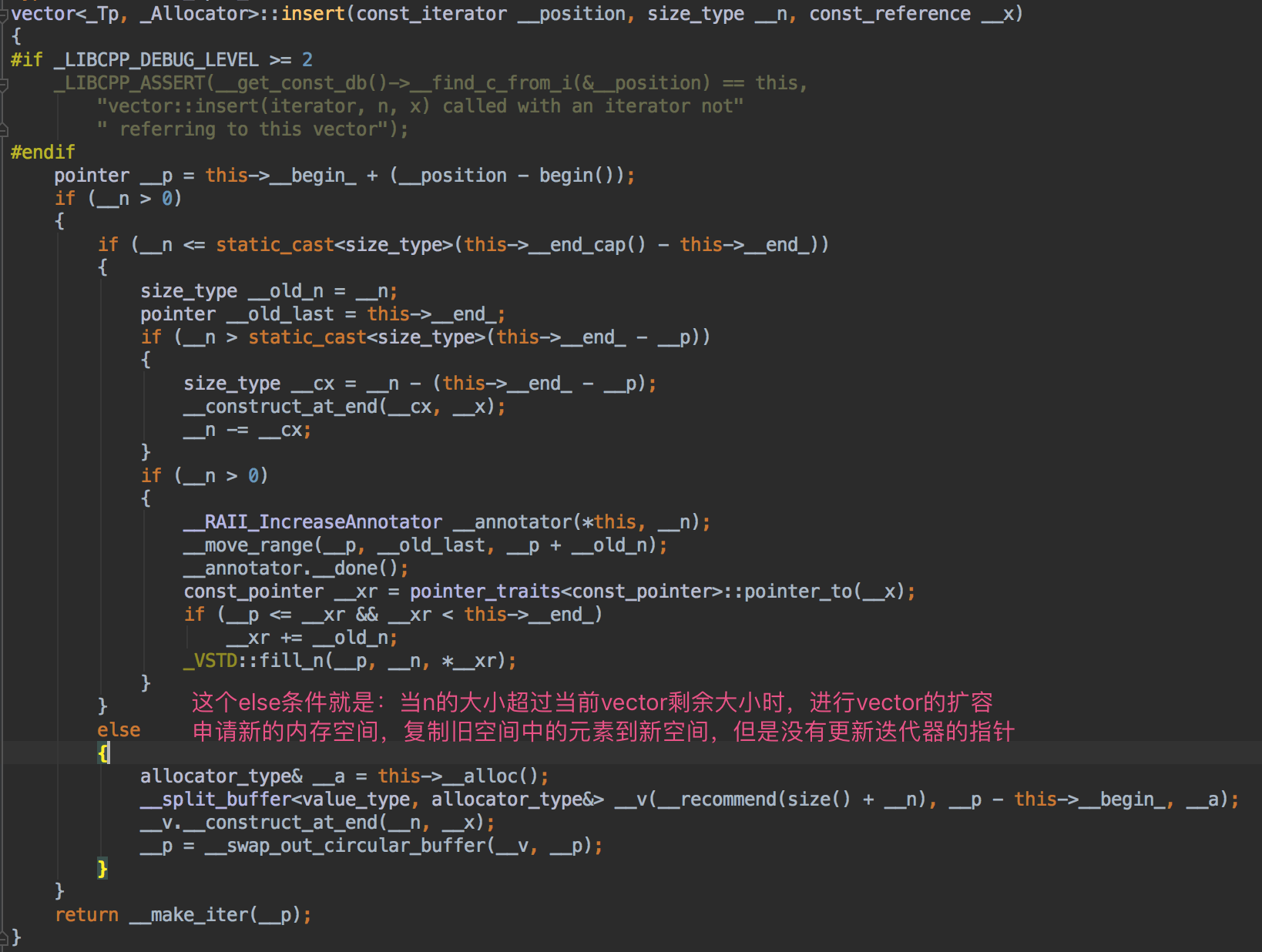# C++ STL迭代器原理和实现

2019/04/10 10:10

### 1. 迭代器简介

STL主要由 容器、迭代器、算法、函数对象、和内存分配器 五大部分构成。

### 2. 迭代器的实现原理

1. list类需要有操作迭代器的方法
1. begin/end
2. insert/erase/emplace
2. list类有一个内部类list_iterator
1. 有一个成员变量ptr指向list容器中的某个元素
2. iterator负责重载++、--、*、->等基本操作
3. list类定义内部类list_iterator的类型别名

### 3. 迭代器的简单实现

my_list.h（重要部分有注释说明

//
// Created by wengle on 2020-03-14.
//

#ifndef CPP_PRIMER_MY_LIST_H
#define CPP_PRIMER_MY_LIST_H

#include <iostream>

template<typename T>
class node {
public:
T value;
node *next;
node() : next(nullptr) {}
node(T val, node *p = nullptr) : value(val), next(p) {}
};

template<typename T>
class my_list {
private:
node<T> *tail;
int size;

private:
class list_iterator {
private:
node<T> *ptr; //指向list容器中的某个元素的指针

public:
list_iterator(node<T> *p = nullptr) : ptr(p) {}

//重载++、--、*、->等基本操作
//返回引用，方便通过*it来修改对象
T &operator*() const {
return ptr->value;
}

node<T> *operator->() const {
return ptr;
}

list_iterator &operator++() {
ptr = ptr->next;
return *this;
}

list_iterator operator++(int) {
node<T> *tmp = ptr;
// this 是指向list_iterator的常量指针，因此*this就是list_iterator对象，前置++已经被重载过
++(*this);
return list_iterator(tmp);
}

bool operator==(const list_iterator &t) const {
return t.ptr == this->ptr;
}

bool operator!=(const list_iterator &t) const {
return t.ptr != this->ptr;
}
};

public:
typedef list_iterator iterator; //类型别名
my_list() {
tail = nullptr;
size = 0;
}

//从链表尾部插入元素
void push_back(const T &value) {
} else {
tail->next = new node<T>(value);
tail = tail->next;
}
size++;
}

//打印链表元素
void print(std::ostream &os = std::cout) const {
for (node<T> *ptr = head; ptr != tail->next; ptr = ptr->next)
os << ptr->value << std::endl;
}

public:
//操作迭代器的方法
//返回链表头部指针
iterator begin() const {
}

//返回链表尾部指针
iterator end() const {
return list_iterator(tail->next);
}

//其它成员函数 insert/erase/emplace
};

#endif //CPP_PRIMER_MY_LIST_H


test.cpp

//
// Created by wengle on 2020-03-14.
//

#include <string>
#include "my_list.h"

struct student {
std::string name;
int age;

student(std::string n, int a) : name(n), age(a) {}

//重载输出操作符
friend std::ostream &operator<<(std::ostream &os, const student &stu) {
os << stu.name << " " << stu.age;
return os;
}
};

int main() {
my_list<student> l;
l.push_back(student("bob", 1)); //临时量作为实参传递给push_back方法
l.push_back(student("allen", 2));
l.push_back(student("anna", 3));
l.print();

for (my_list<student>::iterator it = l.begin(); it != l.end(); it++) {
std::cout << *it << std::endl;
*it = student("wengle", 18);
}
return 0;
}


### 4. 迭代器失效

// inserting into a vector
#include <iostream>
#include <vector>

int main ()
{
std::vector<int> myvector (3,100);
std::vector<int>::iterator it;

it = myvector.begin();
it = myvector.insert ( it , 200 );

myvector.insert (it,200,300);
//it = myvector.insert (it,200,300);
myvector.insert (it,5,500); //当程序执行到这里时，大概率会crash
for (std::vector<int>::iterator it2=myvector.begin(); it2<myvector.end(); it2++)
std::cout << ' ' << *it2;
std::cout << '\n';

return 0;
}### 5. 参考资料0 评论
1 收藏
0